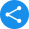# RD Sharma Solutions for Class 6 to 12 Mathematics

## RD Sharma Class 6 to 12 Maths Solutions - Free PDF Download

RD Sharma Solutions will put to rest all your worries and help a student excel in Mathematics. If it is a subject that students are struggling with, these solutions of RD Sharma will guide them step-by-step through the various chapters prescribed in CBSE textbooks. Catered to Class 6 to 12 students, RD Sharma Maths Solutions is categorized according to standards 6 to 12. The Class 6 to 12 RD Sharma Math Solution PDF provides step-by-step solutions to unsolved problems for Class 9 Math textbooks and includes chapters like the Number System, Rationalization, Algebraic Identities, Heron's Formula, and others, while the Class 10 RD Sharma Math Solution PDF provides step-by-step solutions to unsolved problems for Class 10 Math textbooks with chapters related to the Real Numbers, Polynomials, Triangles, Statistics, Quadratic Equations, Circles, Constructions, and others. Chapters on coordinate geometry and congruent triangles are included for both the Class 9 and Class 10 PDFs and if one is confused about the difference between construction and coordinates, you can rest assured that they'll be taken on an extensive and interactive trip through the simplified concepts in these PDFs. The RD Sharma Book Solutions PDF is available for all students across the nation as a free download which can be obtained by visiting Vedantu.com. All you need is basic internet access.

### RD Sharma Solutions for Class 6 to 12 Mathematics

Share this with your friendsShare